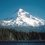1 million dollar prize to prove or disprove the Beal Conjecture

We know many of you are into Fermat's last theorem and thought you might be interested in the Beal Conjecture as well. Expanding the frontier of number theory probably isn't the easiest way to make a million bucks, or even the best reason to work on that kind of problem. That is an impressive prize though, here is the prize page from the American Mathematical Society.Note by Peter Taylor
6 years, 4 months ago

This discussion board is a place to discuss our Daily Challenges and the math and science related to those challenges. Explanations are more than just a solution — they should explain the steps and thinking strategies that you used to obtain the solution. Comments should further the discussion of math and science.

When posting on Brilliant:

• Use the emojis to react to an explanation, whether you're congratulating a job well done , or just really confused .
• Ask specific questions about the challenge or the steps in somebody's explanation. Well-posed questions can add a lot to the discussion, but posting "I don't understand!" doesn't help anyone.
• Try to contribute something new to the discussion, whether it is an extension, generalization or other idea related to the challenge.
• Stay on topic — we're all here to learn more about math and science, not to hear about your favorite get-rich-quick scheme or current world events.

MarkdownAppears as
*italics* or _italics_ italics
**bold** or __bold__ bold

- bulleted
- list

• bulleted
• list

1. numbered
2. list

1. numbered
2. list
Note: you must add a full line of space before and after lists for them to show up correctly
paragraph 1

paragraph 2

paragraph 1

paragraph 2

> This is a quote
This is a quote
# I indented these lines
# 4 spaces, and now they show
# up as a code block.

print "hello world"
# I indented these lines
# 4 spaces, and now they show
# up as a code block.

print "hello world"
MathAppears as
Remember to wrap math in $$...$$ or $...$ to ensure proper formatting.
2 \times 3 $2 \times 3$
2^{34} $2^{34}$
a_{i-1} $a_{i-1}$
\frac{2}{3} $\frac{2}{3}$
\sqrt{2} $\sqrt{2}$
\sum_{i=1}^3 $\sum_{i=1}^3$
\sin \theta $\sin \theta$
\boxed{123} $\boxed{123}$

Sort by:

There are 6(originally 7) unsolved conjectures known as the millennium prize problems and for solving each,the Clay Mathematical Institution offers a million bucks.The Poincare Conjecture is the only one that has been proved.

- 6 years, 4 months ago

thanks a lot for giving such a great problem i'll surely give it a try

- 6 years, 4 months ago

Maybe Fermat proved his last theorem using the Beal equation? (see if you can find out how)

- 6 years, 4 months ago

Wait maybe we can use fermat's little theorem to turn it into a more simpler problem..

- 6 years, 4 months ago